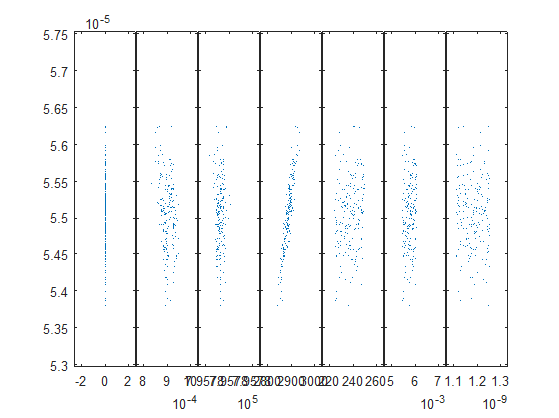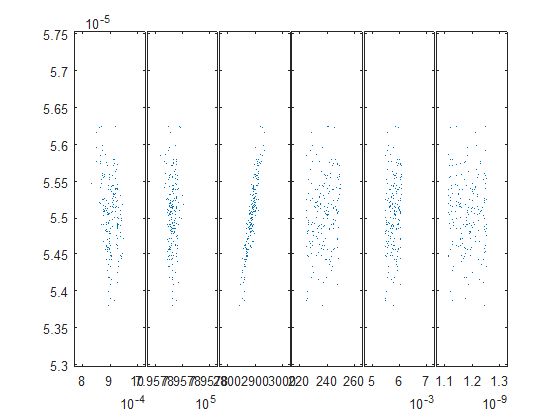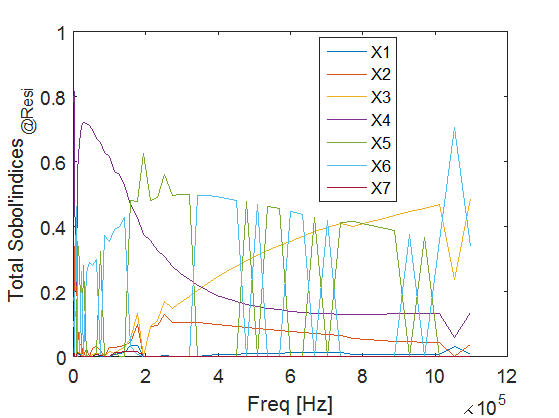# Issues while building PCE surrogate

Hello UQLab community,

I want to use PCE surrogate for the sensitivity analysis … But I’m having a problem while computing PCE coefficeints for my Y1 (or Y2) outputs.
Is there any way to help me figure out why this is not working (see report below)?

Thanks in advance,

— Calculating the PCE coefficients by regression. —
NaN’s cannot be converted to logicals.

Error in uq_PCE_loo_error (line 52)
if ~varY % if the data has 0 variance, set the errors to 0

Error in uq_PCE_OLS_regression (line 49)
[LOO, normEmpErr, optErrorParams] = uq_PCE_loo_error(Psi, M, Y, results.coefficients,
modified_loo);

Error in uq_lar (line 215)
ols_results = uq_PCE_OLS_regression(ones(size(Y,1),1), Y, olsoptions);

Error in uq_PCE_lars (line 64)
lar_results = uq_lar(Psi, Y, lar_options);

Error in uq_PCE_calculate_coefficients_regression (line 197)
lars_results = uq_PCE_lars(univ_p_val, current_model);

Error in uq_PCE_calculate_coefficients (line 47)
uq_PCE_calculate_coefficients_regression(current_model);

Error in uq_calculateMetamodel (line 18)
success = uq_PCE_calculate_coefficients(current_model);

Error in uq_initialize_uq_metamodel (line 358)
success = uq_calculateMetamodel(current_model);

@geofflo

It looks like your data is corrupted, possibly with some NaNs.
You could plot a scatterplot of your input /output matrix `[X Y]` using Matlab `plotmatrix` command (use a Monte Carlo sample to do this, or your data if you pre-computed it) and see if there is nothing obviously wrong.
Best regards

@bsudret

I used pre-computed data in order to build my PCE surrogate for a wide rande of frequencies (frequential behavior).
By plotting the input/output matrix for the first point in the frequential range, I don’t encouter any issue (see attachement).

Thank you for you feedback,@geofflo

Your input X1 looks as a constant, meaning zero variance (thus the error).
It should not be used as input of your PCE construction, or you should declare it as a constant in your `INPUT` object. Did you check that your `INPUT` marginals as declared in UQLab are consistent with the data plotted above?
Best regards

1 Like

@bsudret

Even though I discard X1 component of my input, I meet the same report of error.
I asked to UQLab to detect the marginals for my input data: " Input.Marginals(ll).Type = ‘auto’;
Input.Marginals(ll).Inference.Data = ExpDes_X(:,ll); "

Input object name: Input 1
Dimension(M): 6

Marginals:

## Index | Name | Type | Parameters | Moments

1 | X1 | Gaussian | 9.017e-04, 2.302e-05 | 9.017e-04, 2.302e-05
2 | X2 | Gaussian | 7.958e+05, 6.628e-03 | 7.958e+05, 6.628e-03
3 | X3 | Gaussian | 2.892e+03, 1.794e+01 | 2.892e+03, 1.794e+01
4 | X4 | Uniform | 2.249e+02, 2.500e+02 | 2.375e+02, 7.232e+00
5 | X5 | Uniform | 5.483e-03, 6.094e-03 | 5.789e-03, 1.763e-04
6 | X6 | Uniform | 1.114e-09, 1.257e-09 | 1.185e-09, 4.138e-11

Thanks in advance,Hi @geofflo,

Thanks for creating the post. I think the problem is not related to UQLab but due to the data. As @bsudret mentioned in the previous reply, please double check the model input/output which should contain NaNs.

P.S. You can also upload your code on UQWorld, which will help us better support you.

Best regards

1 Like

Hello @xujia ,

As you mentionned, I find some NaNs in my model output, specifically all 228th elements of the output structure. After fixing theses elements, I succeeded to compute the PCE coefficients. But there are still some warnings (see below) in the UQLab report. Is it normal to receive those warnings?

Thank you in advance for your feedback,

— Calculating the PCE coefficients by regression. —
Warning: Warning: numerical instability!! Gamma for LAR iteration 30 was set to 0 to
prevent crashes.

In uq_lar (line 301)
In uq_PCE_lars (line 64)
In uq_PCE_calculate_coefficients_regression (line 197)
In uq_PCE_calculate_coefficients (line 47)
In uq_calculateMetamodel (line 18)
In uq_initialize_uq_metamodel (line 358)
In uq_core_module/run_initialization_script (line 221)
In uq_core_model/add_module (line 100)
In uq_createModel (line 91)
Warning: Warning: numerical instability!! Gamma for LAR iteration 102 was set to 0 to
prevent crashes.
In uq_lar (line 301)
In uq_PCE_lars (line 64)
In uq_PCE_calculate_coefficients_regression (line 197)
In uq_PCE_calculate_coefficients (line 47)
In uq_calculateMetamodel (line 18)
In uq_initialize_uq_metamodel (line 358)
In uq_core_module/run_initialization_script (line 221)
In uq_core_model/add_module (line 100)
In uq_createModel (line 91)
Warning: Warning: numerical instability!! Gamma for LAR iteration 103 was set to 0 to
prevent crashes.
In uq_lar (line 301)
In uq_PCE_lars (line 64)
In uq_PCE_calculate_coefficients_regression (line 197)
In uq_PCE_calculate_coefficients (line 47)
In uq_calculateMetamodel (line 18)
In uq_initialize_uq_metamodel (line 358)
In uq_core_module/run_initialization_script (line 221)
In uq_core_model/add_module (line 100)
In uq_createModel (line 91)
Warning: Warning: numerical instability!! Gamma for LAR iteration 114 was set to 0 to
prevent crashes.
In uq_lar (line 301)
In uq_PCE_lars (line 64)
In uq_PCE_calculate_coefficients_regression (line 197)
In uq_PCE_calculate_coefficients (line 47)
In uq_calculateMetamodel (line 18)
In uq_initialize_uq_metamodel (line 358)
In uq_core_module/run_initialization_script (line 221)
In uq_core_model/add_module (line 100)
In uq_createModel (line 91)
Warning: Warning: numerical instability!! Gamma for LAR iteration 118 was set to 0 to
prevent crashes.
In uq_lar (line 301)
In uq_PCE_lars (line 64)
In uq_PCE_calculate_coefficients_regression (line 197)
In uq_PCE_calculate_coefficients (line 47)
In uq_calculateMetamodel (line 18)
In uq_initialize_uq_metamodel (line 358)
In uq_core_module/run_initialization_script (line 221)
In uq_core_model/add_module (line 100)
In uq_createModel (line 91)
Warning: Warning: numerical instability!! Gamma for LAR iteration 119 was set to 0 to
prevent crashes.
In uq_lar (line 301)
In uq_PCE_lars (line 64)
In uq_PCE_calculate_coefficients_regression (line 197)
In uq_PCE_calculate_coefficients (line 47)
In uq_calculateMetamodel (line 18)
In uq_initialize_uq_metamodel (line 358)
In uq_core_module/run_initialization_script (line 221)
In uq_core_model/add_module (line 100)
In uq_createModel (line 91)

Below, is the code used for the quantification of uncertainties:

uqlab
Nfreq = 50;
PCE_Resi = cell(Nfreq,1); PCE_Indu = cell(Nfreq,1);
PCESobolAnalysis = cell(Nfreq,1); % structure for PCE models along frequency
for ii = 1:Nfreq
ExpDes_X = [(Re_Zskin{ii})’ (Im_Zskin{ii})’ (Re_Nuprox{ii})’ (Im_Nuprox{ii})’ (Re_Mucore{ii})’ (Im_Mucore{ii})’ (Sigma_core{ii})’];
ExpDes_Y1 = (Resi2{ii})’; ExpDes_Y2 = (Indu2{ii})’;

``````Input = struct;
Input_dim = size(ExpDes_X,2);
for ll = 1:Input_dim
Input.Marginals(ll).Type = 'auto';
Input.Marginals(ll).Inference.Data = ExpDes_X(:,ll);
end
myInput = uq_createInput(Input);
uq_print(myInput);

MetaOpts.Type = 'Metamodel';
MetaOpts.MetaType = 'PCE';
MetaOpts.ExpDesign.X = ExpDes_X;
MetaOpts.ExpDesign.Y = ExpDes_Y1;
MetaOpts.Degree = 2:15;
myPCE_Arb = uq_createModel(MetaOpts);
PCE_Resi{ii} = myPCE_Arb;

% Sensitivity analysis thanks to PCE surrogate
PCESobol.Type = 'Sensitivity';
PCESobol.Method = 'Sobol';
PCESobol.Sobol.Order = 2;
PCESobolAnalysis{ii} = uq_createAnalysis(PCESobol);
``````

end

Dear @geofflo,

Thanks for the feedback. This warning is emitted when there is some numerical instability (usually due to the experimental design) in the LARS iteration. Nevertheless, it does not indicate that the surrogate model is not accurate. To assess the quality of the surrogate model, you check the leave-one-out error (please see this post and the PCE manual for more details).

1 Like

Hello UQLab world,

After solving my PCE construction problem, I computed the Sobol indices from the PCE surrogate over a wide range of frequencies.

Here are the evolutions (see below) of the total Sobol indices from the sensitivity analysis of the 7 inputs (X1, X2, X3, X4, X5, X6, X7) on the two outputs (Resi and Indu). But I am confused when I want to interpret the influences of X5 and X6 as they oscillate and intertwine with each other throughout the frequency.

Have you ever gotten that kind of evolution? If so, how did you interpret such evolution? Or am I visualizing something else instead of what needs to be presented (total Sobol indices instead of the first order indices)?